Open in App
Not now

# Fractions

• Difficulty Level : Expert
• Last Updated : 17 Nov, 2022

Fraction is defined as the part of a whole. Fraction is used not only in mathematics but in real life frequently. The ancient Egyptians were the first people to start using fractions. They used it in their daily life problems, like distributing food and supplies. The word ‘fraction’ is derived from the Latin word ‘fractus’, which means ‘broken’ in English, and it fits right to the meaning of fraction. Let’s learn in detail about fractions and their types.

## What is a Fraction?

Fractions are defined as part of a whole. Fractions are the numerical value that can be a portion or section of any quantity where the quantity can be anything. So, fractions are a section or portion of a quantity. Below is an example of fractions, a pizza is divided into 8 equal parts, and each part is a fraction of the entire pizza, so the parts are the fractions of the pizza, which is the quantity (whole). If one part is taken out, it is represented as 1/8 in fraction form. A fraction can be written in many ways,

• One-Eighth
• 1/8
• 1 by 8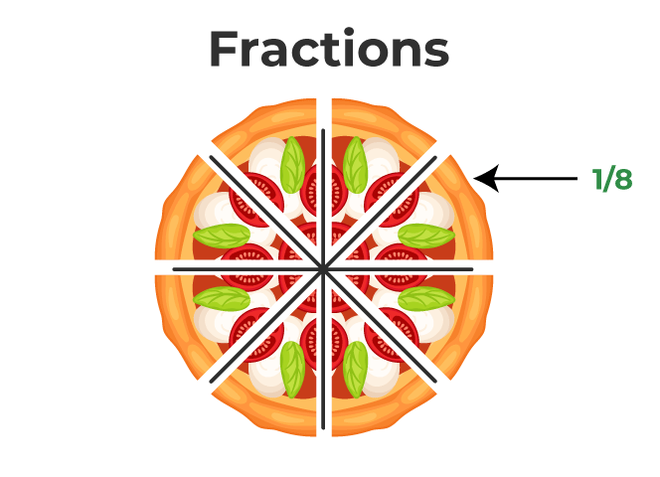The remaining parts collectively will be 7/8 of the pizza as of now; there are only 7 pieces left. Similarly, if 2 pieces are taken out, they will be 2/8 of the entire pizza. It can be simplified and written as 1/4.

## Parts of a Fraction

A fraction contains two parts, i.e., numerator and denominator. The number present on the top is known as the numerator and the number present on the bottom is known as the denominator. The numerator part defines how many parts of the whole are there, whereas the denominator defines the total number of equal parts in a whole. The numerator and denominator are divided by a fractional bar.

• Numerator: The numerator represents the section of the fraction. It is the upper part of the fraction.
• Denominator: The denominator represents the whole in which the fraction is divided. It is the lower part of the fraction.

## Operations on Fractions

Operations on fractions are simple arithmetic operations like addition, multiplication, division, etc. Let’s take a look at the operations that we can do on fractions:

### Reduction of Fractions

Reducing fractions is simplifying fractions by dividing both numerator and denominator with the same value. It is important to note that dividing them by 1 will not make any change in the fraction.

Example: Reduce the fraction ⇢ 88/42

Solution:

88/42 is an improper fraction and both numerator and denominator are divisible by 2. Dividing them by 2:

88/42 = 44/21

Therefore, the reduced fraction becomes 44/21.

### Multiplication of Fractions

Multiplication of fractions does not require any common denominator. Here, simply the numerator is multiplied by the numerator of the other fraction, and the denominator is multiplied by the denominator. It can also be simplified afterward if the fraction can be reduced. Fractions can also be simplified while multiplying with each other.

Example: How to multiply fractions, fractions 21/5 and 35/12.

Solution:

Multiplying fractions:Addition of fractions is possible only when the denominator is the same, in case the denominators of the fractions are not the same, it is important to bring them to a common denominator. Find the LCM of fractions in order to find the common denominator.

Example: How to add fractions 4/5 and 7/3.

Solution:

Here, the denominators of both fractions are not same, LCM of 3 and 5 is 15,### Division of Fractions

Division of fractions does not require any common denominators. The simplest way to divide fractions is to flip the fraction after the division sign, that is, reciprocate the fraction, and change the sign from division to multiplication. Now, simply apply the multiplication of fractions rules and solve.

Example: How to divide fractions 35/20 and 5/10.

Solution:

Dividing fractions: 35/20 ÷ 7/10

Flipping the fraction after division sign and changing the sign to multiplication sign:

35/20 × 10/7

Reducing the fractions:

7/4 × 10/7 = 70/28 = 10/4 = 5/2

### Subtraction of Fractions

Subtraction of fractions is possible only when the denominator is the same. In case the denominators of the fractions are not the same, it is important to bring them to a common denominator. Find the LCM of fractions in order to find the common denominator.

Example: Subtract fractions 1/2 from 3/5.

Solution:

The least common denominator of 2 and 5 is 10. Solving:## Properties of Fractions

There are some important properties of fractions similar to whole numbers, natural numbers, etc. Let’s take a look at those properties:

• For the Addition of fractions and multiplication of fractions, commutative and associative properties are true.
• Fractional multiplication gives 1, and the identity element is 0.
• The multiplicative inverse property reciprocates the fraction. That is, a/b is b/a, where a and b are non-zero numbers.
• The distributive property of multiplication over addition holds true for fractions.

## Types of Fractions

There are majorly four types of fractions. They are unit fractions, proper fractions, improper fractions, and mixed fractions. They are categorized on the basis of their numerator and denominator. Let’s learn about all four types in detail:

### Unit Fraction

A unit fraction is defined as a fraction with 1 as a numerator and is known as a Unit Fraction, for example – 1/8, 1/10, 1/4, 1/6, 1/11, etc. It can be said that all unit fractions are proper fractions since all unit fractions have 1 in the numerator, which is lesser than the denominator. The below given example explains unit fraction very nicely. Here, a cake is divided into 4 equal parts. If the whole cake is 1, then each quarter of the cake is the fraction 1/4.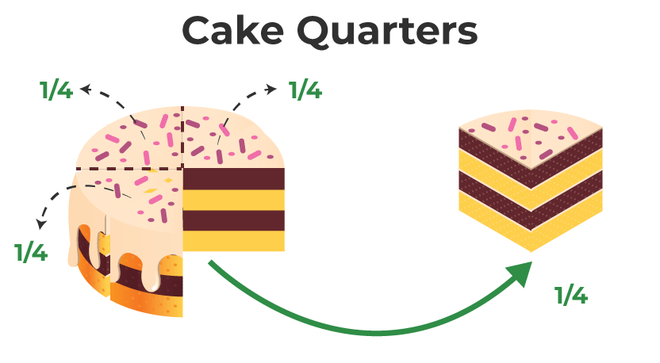### Proper Fraction

A Proper fraction is defined as a fraction in which the numerator value is less than the denominator value then. It is known as a proper fraction, for example, 4/9, 1/10, 2/5, 3/7, 5/9, etc.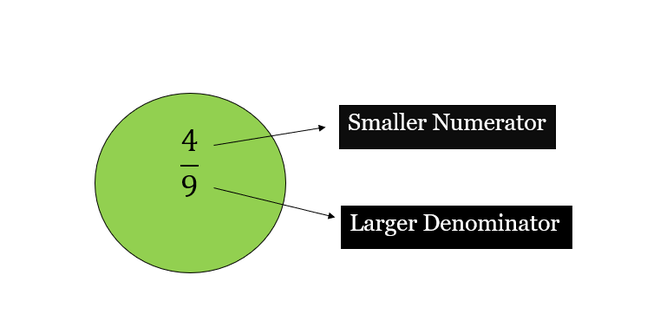### Improper Fraction

An Improper fraction is defined as a fraction in which the numerator value is greater than the denominator value, then it is known as an improper fraction, for example, 6/5, 11/10, 11/5, 5/3, 2/1, etc.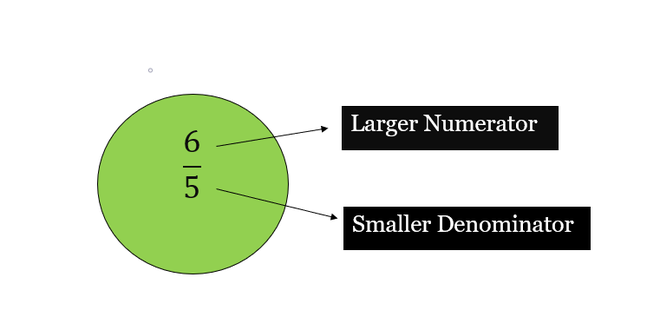### Mixed Fraction

A Mixed fraction consists of a whole number with a proper fraction then, it is known as a mixed fraction. For Example, if 2 is a whole number and 1/4 is a fraction, then 2¼ is a mixed fraction.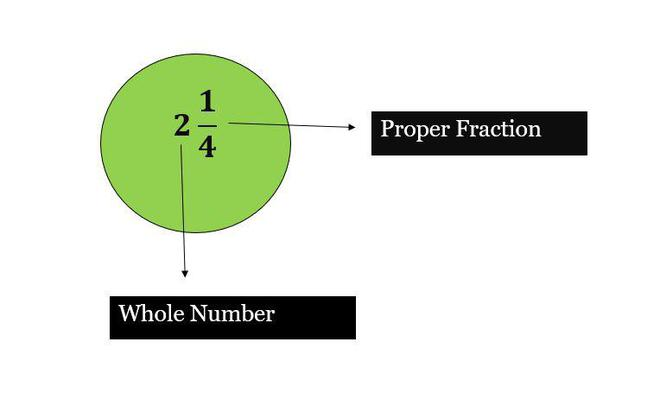## Other Types of Fractions

Other types of fractions are on the basis of groups. They involve like and unlike fractions and equivalent fractions. All three types of fractions are explained below in complete detail.

### Like Fractions

Two or more than two fractions whose denominators are the same are known as like fractions. Examples of like fractions are,

1. 2/9, 3/9, 5/9, 9/9, 4/9 (Here, the denominators of all the fractions are the same, that is, 9).
2. 3/10, 7/10, 1/10, 9/10, 6/10 (Here, the denominators of all the fractions are the same, that is, 10).
3. 1/7, 2/7, 4/7, 5/7, 7/7, 8/7.
4. 1/2, 7/2, 6/2, 5/2, 9/2.
5. 7/5, 1/5, 4/5, 3/5.

### Unlike Fractions

The fractions with different denominators are known as unlike fractions. Example of, unlike fractions,

1. 9/2, 1/6, 5/4, 7/3 (Here, the denominator of all the fractions is different).
2. 1/2, 1/4, 2/3, 5/6, 8/9 (Here, the denominator of all the fractions is different).
3. 3/8, 2/3, 3/5, 2/7.
4. 1/9, 2/7, 3/4, 2/5, 3/2.
5. 4/2, 1/6, 2/3, 7/5.

### Equivalent Fractions

Equivalent fractions are defined as those fractions which result in the same value after simplification, and then they are equivalent to each other. Solving equivalent fractions can be done by either multiplying both the numerator and denominator by the same number or dividing the numerator and denominator by the same number. Below are some examples of equivalent fractions: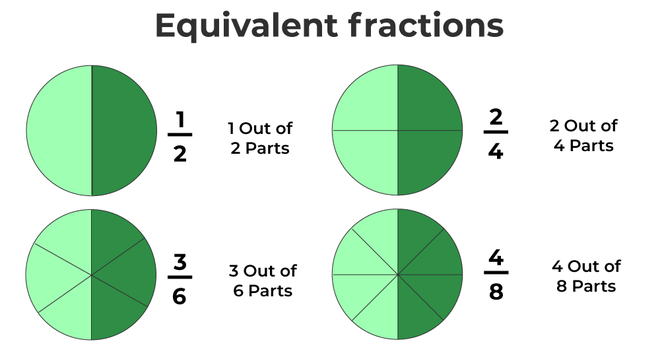Example: Find two equivalent fractions of 4/12.

Solution:

Equivalent fraction by multiplying with the same number, lets multiply by 2:

(4 × 2)/(12 × 2)

= 8/24

Equivalent fraction by division with the same number, here, both numerator and denominator are divisible by 4, dividing by 4:

(4 ÷ 4)/(12 ÷ 4)

= 1/3

## Fraction on a Number Line

Fractions on a number line are represented between the interval of two integers. Fractions are a part of the whole. Therefore, the whole is divided into equal parts, the number of parts between the integers is decided by the denominator value, and the numerator value is the point where the fraction lies. For example, if we are required to represent 1/8 on a number line, the division will be between 0 and 1, and since 8 is in the denominator, the whole is divided into 8 equal parts, where the first represents 1/8. Similarly, the second part represents 2/8, and so on.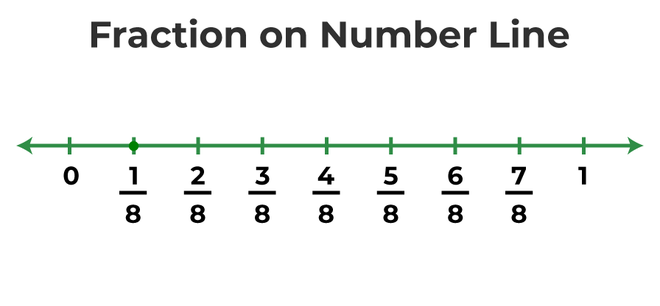## How to Convert Fractions into Decimals?

Decimals are the numbers that represent fractions but in decimal form. For instance, 1/4 in fraction can be written as 0.25 in decimal form. Decimals are mostly preferred as they can be used for multiple mathematical operations where solving fractions seems complex, like addition, subtraction, etc. For example: In order to add 1/2 and 1/4, it is easier to solve the terms in decimals. Converting fractions into decimals ⇢ 0.5 + 0.25 = 0.75.

## How to Convert a Decimal into a Fraction?

Decimals are converted into decimals as sometimes mathematical operations are easily solved in fraction form. Mathematical operations like multiplication and division are sometimes easier with fractions. Following are the steps that can be followed to convert a decimal into a fraction:

• Convert the decimal into p/q form where q = 1.
• Now multiply the numerator and denominator by 10 till the numerator becomes a whole number from decimals. For example, if there are two numbers after the decimal point, multiply 100/100.
• Now, simplify the fraction obtained.

## Value of Pi in Fraction

Pi (π) is a mathematical constant that is defined as the ratio of a circle’s circumference to its diameter. The value of pi in decimal is approximately equal to 3.14. The value of pi (π) in fractions is 22/7. The value of pi is used both in decimal and fraction form based on the type of question given.

For example: Convert 0.8 into a fraction.

• Convert into p/q form ⇢ 0.8/1
• Multiply 10/10 ⇢ 0.8/1 × 10/10
• Simplifying fraction ⇢ 8/10 = 4/5.

## Solved Examples on Fractions

Question 1: Write two equivalent fractions of 3/39.

Solution:

Equivalent fraction by multiplying with the same number, lets multiply by 2:

(3 × 2)/(39 × 2)

= 6/78

Equivalent fraction by division with the same number, here, both numerator and denominator are divisible by 3, dividing by 3:

(3 ÷ 3)/(39 ÷ 3)

= 1/13

Question 2: In a class of 90 students, 1/3rd of the students do not like cricket. How many students like cricket?

Solution:

Fraction of students that do not like cricket = 1/3

Fraction of student that like cricket = 1 – 1/3

= (3 – 1)/3

= 2/3rd students like cricket.

Number of students that like cricket = 2/3 × 90

= (2 × 30)

= 60

Therefore, 60 students like cricket.

Question 3: What type of fraction is this – 1/2, 1/5, 1/7, 1/10, 1/3?

This is a Unit fraction because all the fractions have 1 as a numerator.

Question 4: Determine the type of fraction 7¼.

Mixed fraction because it is a combination of both whole number and a proper fraction in which 7 is the whole number and 1/4 is the proper fraction.

Question 5: What is the equivalent fraction of 7/5?

To find the equivalent fraction of 7/5, we need to multiply both numerator and denominator by the same number.

Hence, (7/5) × (2/2) = 14/10

Therefore equivalent fraction of 7/5 is 14/10.

## FAQs on Fractions

Question 1: What is comparing fractions?

Comparing fractions is done to understand which fraction is larger or which fraction is smaller among the given fractions. If the denominator of the fractions given are same, only the numerators are compared, if the fractions have different denominators, first the LCM of fractions are taken, and then the numerators are compared. For example, 3/19 and 7/19 when compared, it can be observed that the denominator are same, therefore, numerators are compared, 3 > 7, therefore, 7/19 > 3/19.

Question 2: How to multiply fractions?

Multiplication of fraction do not require any common denominator. Here, simply the numerator is multiplied by numerator of the other fraction and denominator is multiplied by the denominator. It can also be simplified afterwards if the fraction can be reduced. Fractions can also be simplified while multiplying with each other. For example: 3/7 × 1/2 = 3/14.

Question 3: How to divide fractions?

Division of fractions do not require any common denominators, the simplest way to divide fractions is to flip the fraction after division sign, that is, reciprocate the fraction, and change the sign from division to multiplication. Now, simply apply the multiplication of fractions rules and solve. For example: 4/9 ÷ 2/3 = 4/9 × 3/2 = 2/3.

Question 4: How to add fractions?

Adding fractions is possible only when the denominator are same, incase the denominators of the fractions are not same, it is important to bring them to a common denominator. Find the LCM of fractions in order to find the common denominator. For example: 3/4 + 1/2 = 3/4 + 2/4 = 5/4.

Question 5: What is an improper fraction?

An Improper fraction is defined as a fraction in which the numerator value is greater than the denominator value, then it is known as an improper fraction, for example, 7/5, 13/10, 9/5, 17/3, 5/1, etc.

Question 6: What fraction of a day is 8 hours?

We know that 1 day has 24 hours.

1 hour = 1/24 days

8 hours = 1/24 × 8 days

8 hours = 8/24 = 1/3 days

Therefore, 8 hours has 1/3 days.

### Related Articles

My Personal Notes arrow_drop_up
Related Articles